# Problem-2 (Basic CVP analysis, CVP graph or break even chart, break-even analysis)

Beta company sells blouses in Washington, USA. Blouses are imported from Pakistan and are sold to customers in Washington at a profit. Salespersons are paid basic salary plus a decent commission of \$14 on each sale made by them. Selling price and expense data is given below: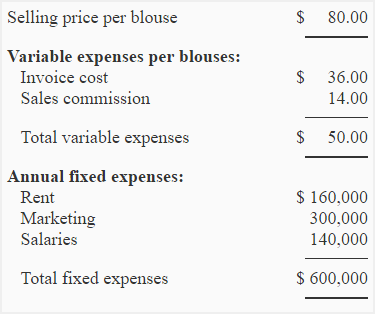Required:

1. Compute the break-even point in units and in dollars using the information given above.
2. Prepare a CVP graph (break-even chart) and show the break-even point on the graph.
3. What would be net operating income or loss if company sells 18,500 blouses in a year?
4. If the manage is paid a commission of \$6 blouse (in addition to the salesperson’s commission), what will be the effect on company’s break-even point?
5. As an alternative to (3) above, company is thinking to pay \$6 commission to manager on each blouse sold in excess of break-even point. What will be the effect of these changes on the net operating income or loss of the Beta company if 23,500 blouses are sold in a year?
6. Refer to the original data. What will be the break-even point of the company if commission is entirely eliminated and salaries are increased by \$214,000?

## Solution:

### (1) Calculation of break-even point:

a. Equation method:

SpQ = VeQ + Fe

\$80Q = \$50Q + \$600,000

\$80Q – \$50Q = \$600,000

\$30Q = \$600,000

Q = \$600,000/\$30

Q = 20,000 blouses

20,000 blouses × \$80.00 per blouse = \$1,600,000

b. Contribution margin method:

Break-even point = Fixed expenses/Contribution margin per unit

= \$600,000/\$30*

= 20,000 blouses

20,000 blouses × \$80.00 per blouse = \$1,600,000

*\$80 – \$50 = \$30

Alternatively;

Break-even point = Fixed expenses/CM ratio

= \$600,000/0.375*

= \$1,600,000

*\$30/\$80 = 0.375

### (2) CVP graph or break-even chart: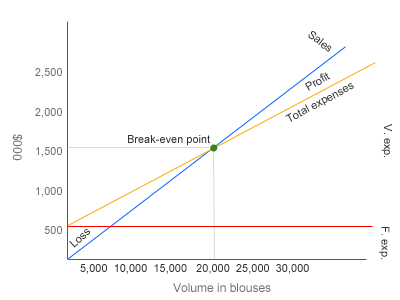### (3). Net operating income or loss if 18,500 blouses are sold in a year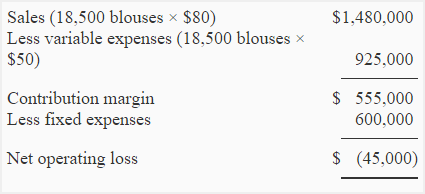An alternative and more simple approach is given below: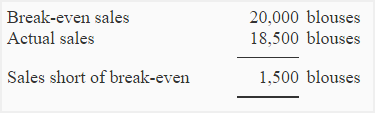Net operating loss = Sales short of break-even × Contribution margin per unit

= 1,500 blouses × \$30

= \$45,000

### (4) Break-even point if manager is also paid a commission of \$6 per blouse sold:

The payment of a commission of \$6 to manager will increase variable expenses and decrease contribution margin. Now the variable expenses will be \$56 (\$50 + \$6) per unit and contribution margin will be \$24 (\$80 – \$56) per unit.

a. Equation method:

SpQ = VeQ + Fe

\$80Q = \$56Q + \$600,000

\$80Q – \$56Q = \$600,000

\$24Q = \$600,000

Q = \$600,000/\$24

Q = 25,000 blouses

25,000 blouses × \$80.00 per blouse = \$2,000,000

b. Contribution margin method:

Break-even point in units = Fixed expenses/Contribution margin per unit

\$600,000/\$24*

25,000 blouses

25,000 blouses × \$80.00 per blouse = \$2,000,000

*\$80 – \$56 = \$24

Alternatively;

Break-even point = Fixed expenses/CM ratio

= \$600,000/0.30*

= \$2,000,000

*\$24/\$80 = 0.30

### (4) Effect on net operating income or loss if manager is paid a commission of \$6 on each blouse sold after break-even point: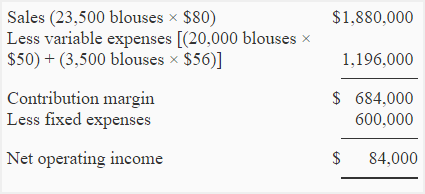Alternatively the net operating income of \$84,000 may also be computed by using the following simple approach: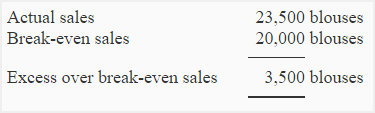3,500 shirts × \$24 per shirt* = \$84,000 profit

*[\$80 – (\$50 + \$6)] = \$24

### (5) Break-even point after elimination of commission and increase in salaries:

The new variable expenses are \$36 (invoice cost, no commission) and new fixed expenses are \$814,000 (\$600,000 + \$214,000).

a. Equation method:

SpQ = VeQ + Fe

\$80Q = \$36Q + \$814,000

\$80Q – \$36Q = \$814,000

\$44Q = \$814,000

Q = \$814,000/\$44

Q = 18,500 blouses

18,500 blouses × \$80.00 per blouse = \$1,480,000

b. Contribution margin method:

= \$814,000/\$44*

= 18,500 blouses

18,500 blouses × \$80 = \$1,480,000

*\$80 – \$36 = \$44

Alternatively;

Break-even point = Fixed expenses/CM ratio

= \$814,000/0.55*

= \$1,480,000

*\$44/\$80 = 0.55

Show your love for us by sharing our contents.

### 3 Comments on Problem-2 (Basic CVP analysis, CVP graph or break even chart, break-even analysis)

1.gordon

She can rent an exclusive site for R300 a day, payable in cash. This site can be rented for 10 hours per day. The market is selling hamburgers at R15 each. she will do well if she can sell a better quality double cheese burger at the same price. further decided to rent a fully equipped trailer, custom made for a hamburger stall with all additional accessories e.g. signboards, gas rent is R460 per day. she would like to make a profit of R500 per day plus an additional R240 per day. Further found that the ingredients of 1 hamburger ad up to exactly R5. She also decided to make a sign for the trailer for R500.

How many hamburgers must she sell per day to cover her cost?

Motivate the answer by proving it with a secondary calculation Use the below diagram to work

hamburgers Fixed cost Cost/Unit Selling Price Income Variable cost Total cost

0

50

100

150

200

250

300

1.gordon

please can i be help with the above please…..

2.rubel barua

variable cost unit law?